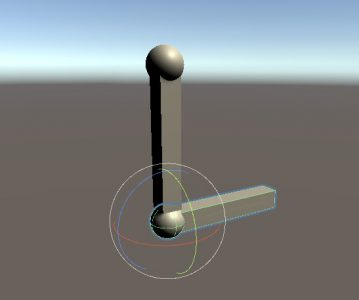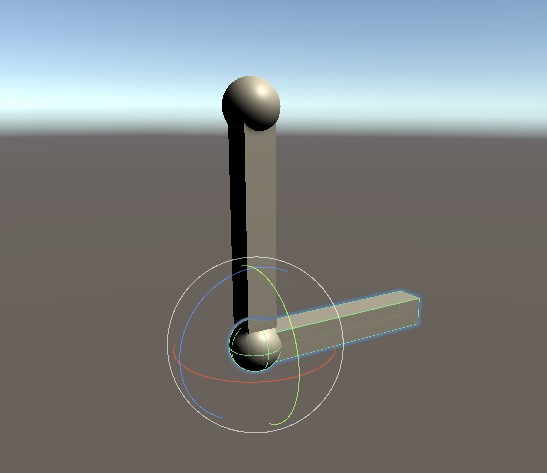# Quaternion 掃盲

## 取得單一 Quaternion 的某個方向(Direction – vector)?

“它” Quaternion 自己就是代表一個物件的轉向 (Local space).

```Quaternion facing = /* whatever */;
Vector3 myForward = facing * Vector3.forward;
Vector3 myRight = facing * Vector3.right;
Vector3 myUpward = facing * Vector3.up;
Vector3 myLeft = -myRight;
Vector3 myBack = -myForward;
Vector3 myBottom = -myUpward;```

## 四元數的轉向疊加 (相乘)“上臂” 的轉動會帶動 “前臂”

“下臂” 是子層級的轉向.

```Quaternion upperArm = /* local downward */
Quaternion lowerArm = /* local upward */

Quaternion armRotation = upperArm * lowerArm;```### 多層轉向消除/減法 (誤)

Quaternion answer = A * Quaternion.Inverse(B);

### 相乘順序的特性

“前臂”先轉向上方, 然後”上臂”才轉到下方.

Quaternion parent; // 父層轉向
Quaternion child; // 子層轉向
Quaternion combine; // 最終轉向

1) combine = parent * child;

2) child == parent.Inverse() * combine;

3) parent == combine * child.Inverse();

Reference :

https://en.wikipedia.org/wiki/Quaternions_and_spatial_rotation